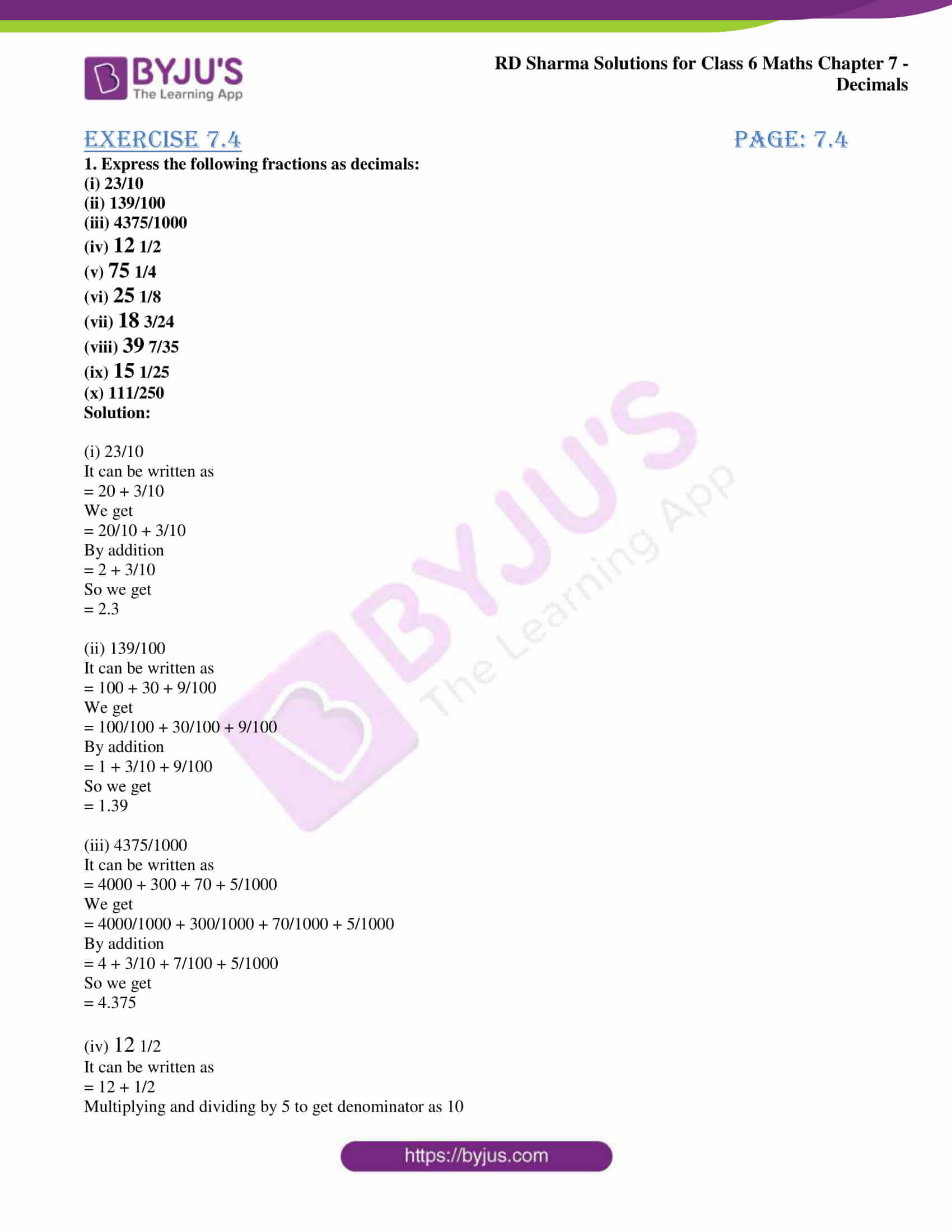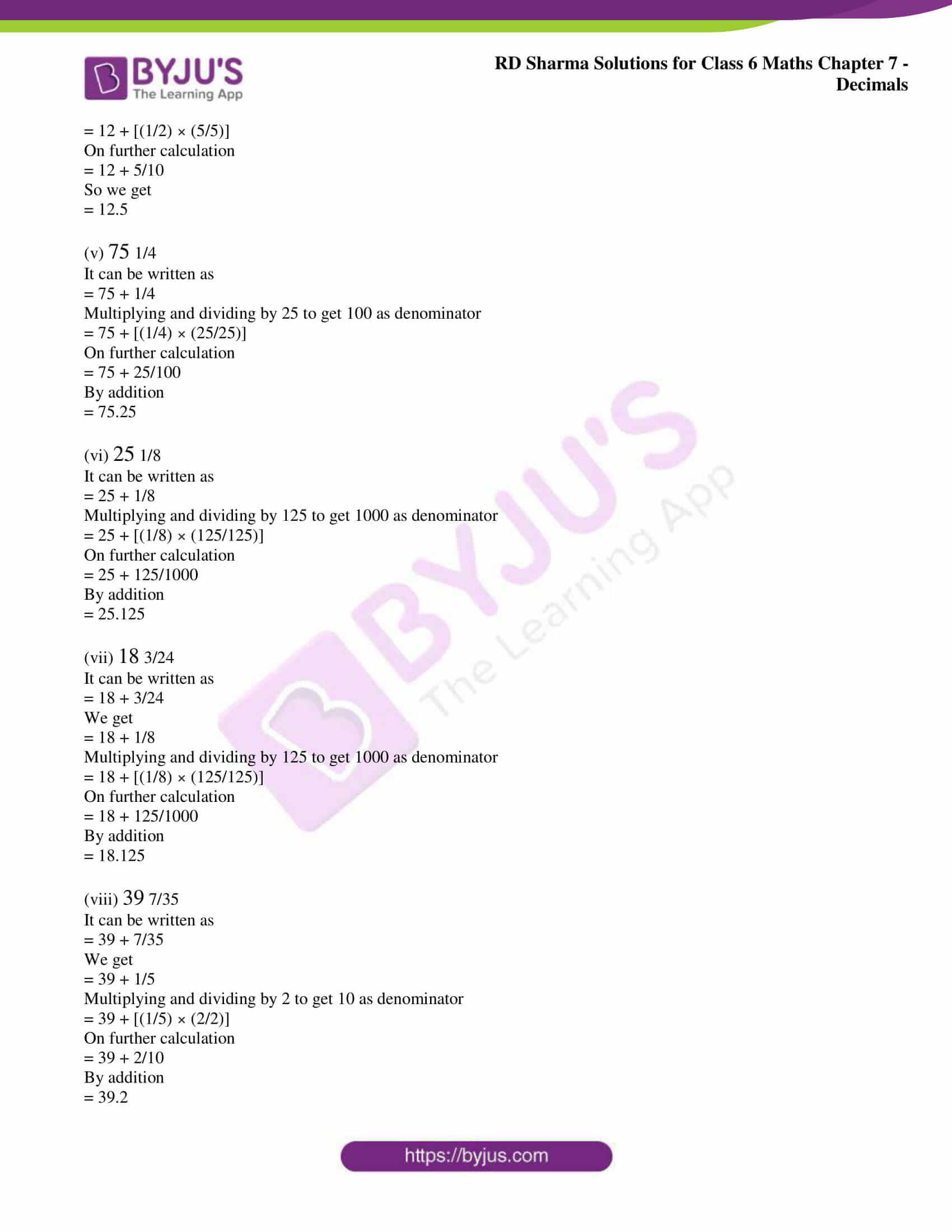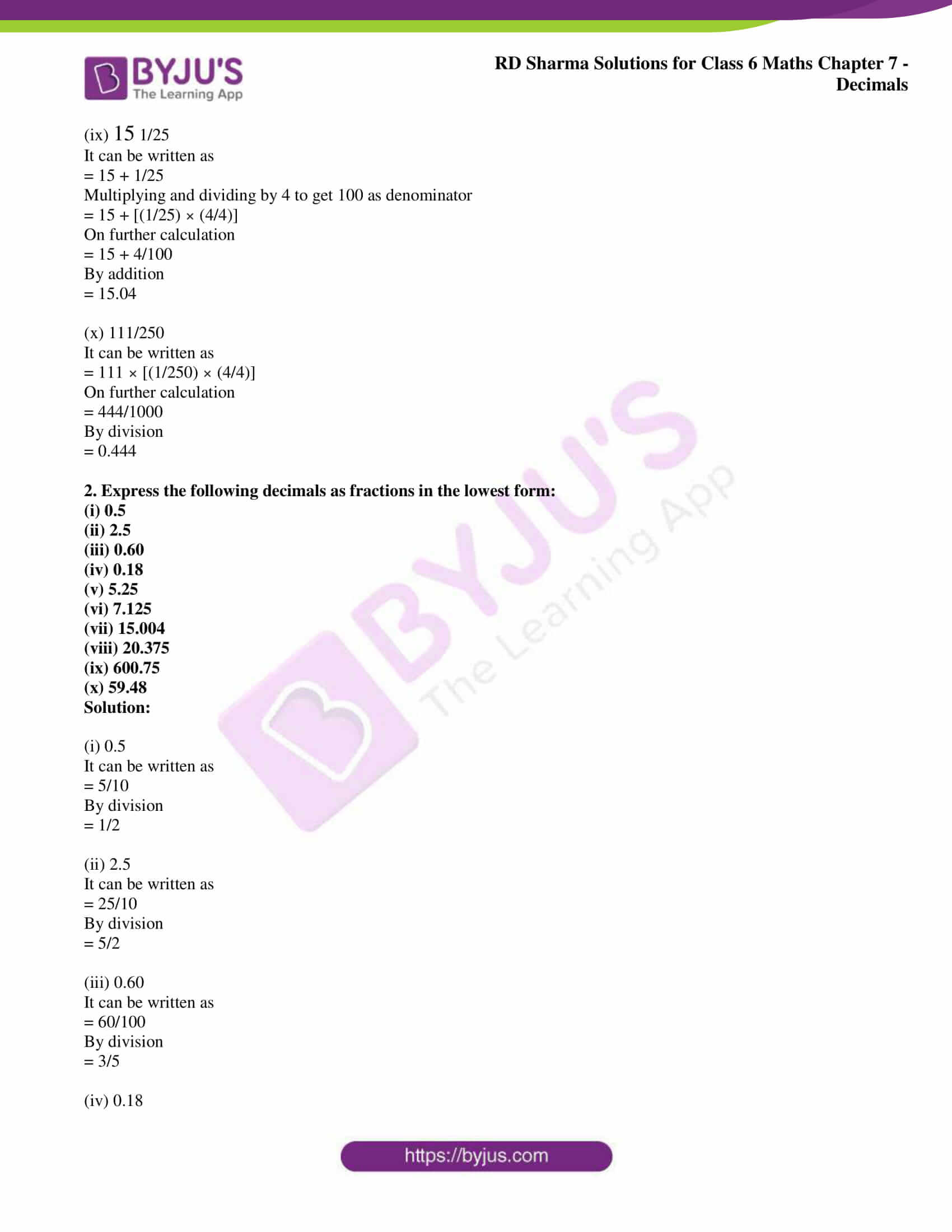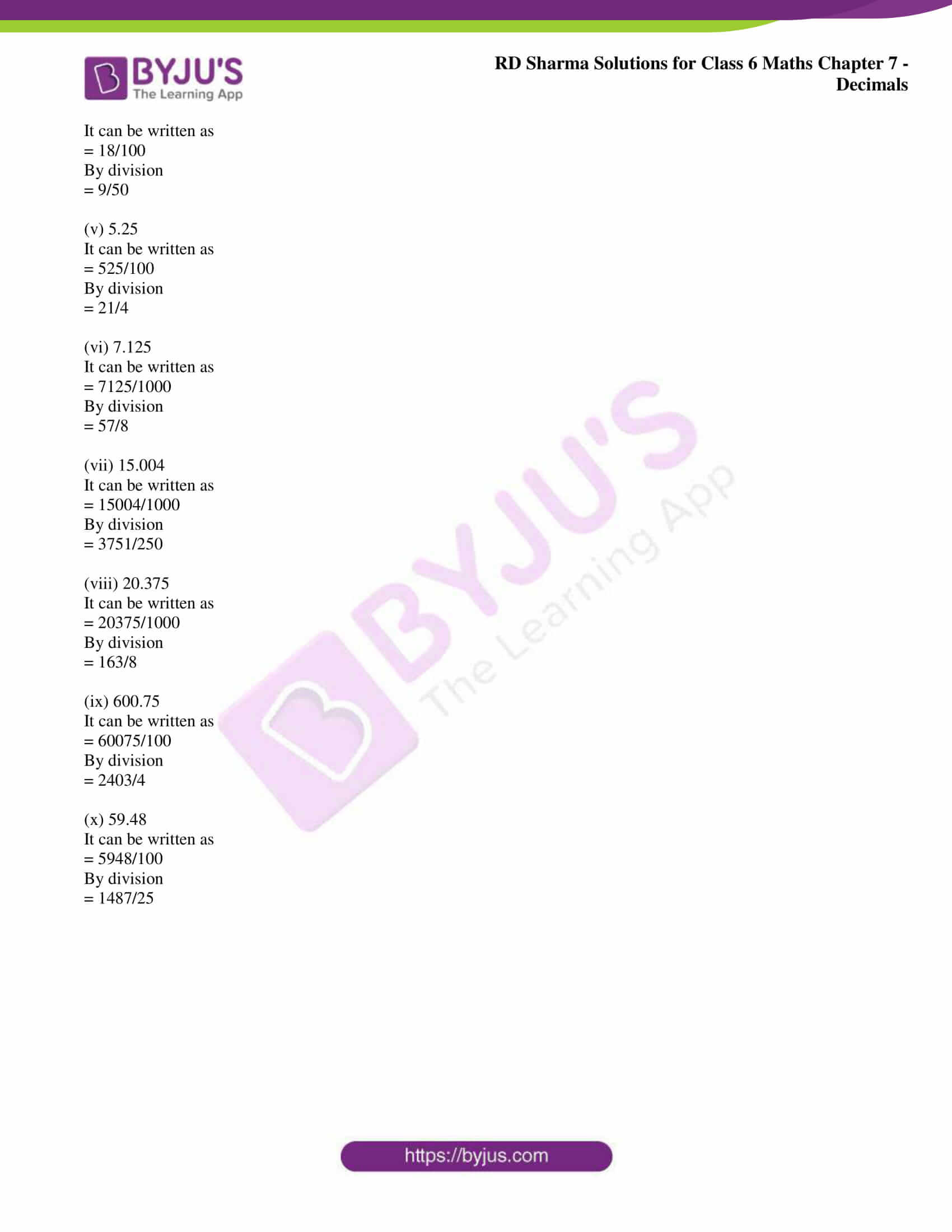# RD Sharma Solutions for Class 6 Chapter 7: Decimals Exercise 7.4

Learning Mathematics is made fun for the students with the help of RD Sharma Solutions Class 6. Exercise 7.4 covers major concepts like steps followed to convert the given fractions as decimals and vice versa. The fractions can be expressed as decimals using 10, 100 and 1000 as the denominator. Students gain a better hold on the concepts covered in Chapter 7 using RD Sharma Solutions for Class 6 Maths Chapter 7 Decimals Exercise 7.4 PDF which are provided here.

## RD Sharma Solutions for Class 6 Chapter 7: Decimals Exercise 7.4 Download PDF### Access RD Sharma Solutions for Class 6 Chapter 7: Decimals Exercise 7.4

1. Express the following fractions as decimals:

(i) 23/10

(ii) 139/100

(iii) 4375/1000

(iv) 12 1/2

(v) 75 1/4

(vi) 25 1/8

(vii) 18 3/24

(viii) 39 7/35

(ix) 15 1/25

(x) 111/250

Solution:

(i) 23/10

It can be written as

= 20 + 3/10

We get

= 20/10 + 3/10

= 2 + 3/10

So we get

= 2.3

(ii) 139/100

It can be written as

= 100 + 30 + 9/100

We get

= 100/100 + 30/100 + 9/100

= 1 + 3/10 + 9/100

So we get

= 1.39

(iii) 4375/1000

It can be written as

= 4000 + 300 + 70 + 5/1000

We get

= 4000/1000 + 300/1000 + 70/1000 + 5/1000

= 4 + 3/10 + 7/100 + 5/1000

So we get

= 4.375

(iv) 12 1/2

It can be written as

= 12 + 1/2

Multiplying and dividing by 5 to get denominator as 10

= 12 + [(1/2) × (5/5)]

On further calculation

= 12 + 5/10

So we get

= 12.5

(v) 75 1/4

It can be written as

= 75 + 1/4

Multiplying and dividing by 25 to get 100 as denominator

= 75 + [(1/4) × (25/25)]

On further calculation

= 75 + 25/100

= 75.25

(vi) 25 1/8

It can be written as

= 25 + 1/8

Multiplying and dividing by 125 to get 1000 as denominator

= 25 + [(1/8) × (125/125)]

On further calculation

= 25 + 125/1000

= 25.125

(vii) 18 3/24

It can be written as

= 18 + 3/24

We get

= 18 + 1/8

Multiplying and dividing by 125 to get 1000 as denominator

= 18 + [(1/8) × (125/125)]

On further calculation

= 18 + 125/1000

= 18.125

(viii) 39 7/35

It can be written as

= 39 + 7/35

We get

= 39 + 1/5

Multiplying and dividing by 2 to get 10 as denominator

= 39 + [(1/5) × (2/2)]

On further calculation

= 39 + 2/10

= 39.2

(ix) 15 1/25

It can be written as

= 15 + 1/25

Multiplying and dividing by 4 to get 100 as denominator

= 15 + [(1/25) × (4/4)]

On further calculation

= 15 + 4/100

= 15.04

(x) 111/250

It can be written as

= 111 × [(1/250) × (4/4)]

On further calculation

= 444/1000

By division

= 0.444

2. Express the following decimals as fractions in the lowest form:

(i) 0.5

(ii) 2.5

(iii) 0.60

(iv) 0.18

(v) 5.25

(vi) 7.125

(vii) 15.004

(viii) 20.375

(ix) 600.75

(x) 59.48

Solution:

(i) 0.5

It can be written as

= 5/10

By division

= 1/2

(ii) 2.5

It can be written as

= 25/10

By division

= 5/2

(iii) 0.60

It can be written as

= 60/100

By division

= 3/5

(iv) 0.18

It can be written as

= 18/100

By division

= 9/50

(v) 5.25

It can be written as

= 525/100

By division

= 21/4

(vi) 7.125

It can be written as

= 7125/1000

By division

= 57/8

(vii) 15.004

It can be written as

= 15004/1000

By division

= 3751/250

(viii) 20.375

It can be written as

= 20375/1000

By division

= 163/8

(ix) 600.75

It can be written as

= 60075/100

By division

= 2403/4

(x) 59.48

It can be written as

= 5948/100

By division

= 1487/25